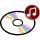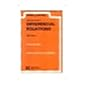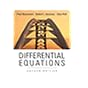Dr. S. R. Lasker Library Online Catalogue

Normal view

# Differential equations / Paul Blanchard, Robert L. Devaney, Glen R. Hall.

Material type:TextLanguage: English Publication details: c2002. Edition: 2nd edDescription: xx, 786 p. : ill. ; 24 cmISBN: 0534385141; 9780534385149; 0534228585 Subject(s): Differential equationsDDC classification: 515.35 LOC classification: QA371 | .B63 2002Online resources: WorldCat details
Contents:
TOC 1 First-order differential equations -- Modeling via differential equations -- Analytic technique: separation of variables -- Qualitative technique: slope fields -- Numerical techniques: Euler's method -- Existence and uniqueness of solutions -- Equilibria and the phase line -- Bifurcations -- Linear differential equations -- Changing variables -- Labs for chapter 1 -- 2 First order systems -- Modeling via systems -- The geometry of systems -- Analytic methods for special systems -- Euler's method for systems -- The Lorenz equations -- Labs for chapter 2 -- 3 Linear systems -- Properties of linear systems and linearity principle -- Straight-line solutions -- Phase planes for linear systems with Eigenvalues -- Complex Eigenvalues -- Special cases: repeated and Eigenvalues -- Second-order linear equations -- The trace-determinant plane -- Linear systems in three dimensions -- 4 Forcing and resonance -- Forced harmonic oscillators -- Sinusoidal forcing -- Undamped forcing and resonance -- Amplitude and phase of the steady state -- The Tacoma Narrows Brodge 5 Nonlinear systems -- Equilibrium point analysis -- Qualitative analysis -- Hamiltonian systems -- Dissipative systems -- Nonlinear systems in three dimensions -- Periodic forcing of nonlinear systems and chaos -- Labs for chapter 5 -- 6 Laplace Transformes -- Laplace Transforms -- Discontinuous functions -- Second-order equations -- Delta functions and impulse forcing -- Convolutions -- The qualitative theory of Laplace Transforms -- Labs for chapter 6 -- 7 Numerical methods -- Numerical Error in Euler's Method -- Improving Euler's method -- The Runge-Kutta method -- The effects of finite arithmetic -- Labs for chapter 7 -- 8 Discrete dynamical systems -- the discrete logistic equation -- Fixed points and periodic points -- Bifurcations -- Chaos -- Chaos in the Lorenz system -- Labs for chapter 8.
Tags from this library: No tags from this library for this title.
Star ratingsAverage rating: 0.0 (0 votes)
Holdings
Item type Current library Collection Call number Copy number Status Date due Barcode Item holdsText
Reserve Section
Non-fiction 515.35 BLD 002 (Browse shelf(Opens below)) C-1 Not For Loan 17106CDs & DVDs
Audio Visual
Non-fiction 515.35 BLD 2002 (Browse shelf(Opens below)) C-1 Available CD-909
Total holds: 0
##### Browsing EWU Library shelves, Shelving location: Reserve Section Close shelf browser (Hides shelf browser)
 No cover image available No cover image availableNo cover image available No cover image available515.33 EDD Differential calculus for beginners 515.33 EDD Differential calculus for beginners 515.35 AYS 1972 Schaum's outline of theory and problems of differential equations in SI metric units / 515.35 BLD 002 Differential equations / 515.35 KHD 1992 Differential equations 515.35 KHD 1997 Differential equations 515.35 ROD 1984 Differential equations /

Includes index.

TOC 1 First-order differential equations --
Modeling via differential equations --
Analytic technique: separation of variables --
Qualitative technique: slope fields --
Numerical techniques: Euler's method --
Existence and uniqueness of solutions --
Equilibria and the phase line --
Bifurcations --
Linear differential equations --
Changing variables --
Labs for chapter 1 --
2 First order systems --
Modeling via systems --
The geometry of systems --
Analytic methods for special systems --
Euler's method for systems --
The Lorenz equations --
Labs for chapter 2 --
3 Linear systems --
Properties of linear systems and linearity principle --
Straight-line solutions --
Phase planes for linear systems with Eigenvalues --
Complex Eigenvalues --
Special cases: repeated and Eigenvalues --
Second-order linear equations --
The trace-determinant plane --
Linear systems in three dimensions --
4 Forcing and resonance --
Forced harmonic oscillators --
Sinusoidal forcing --
Undamped forcing and resonance --
Amplitude and phase of the steady state --
The Tacoma Narrows Brodge 5 Nonlinear systems --
Equilibrium point analysis --
Qualitative analysis --
Hamiltonian systems --
Dissipative systems --
Nonlinear systems in three dimensions --
Periodic forcing of nonlinear systems and chaos --
Labs for chapter 5 --
6 Laplace Transformes --
Laplace Transforms --
Discontinuous functions --
Second-order equations --
Delta functions and impulse forcing --
Convolutions --
The qualitative theory of Laplace Transforms --
Labs for chapter 6 --
7 Numerical methods --
Numerical Error in Euler's Method --
Improving Euler's method --
The Runge-Kutta method --
The effects of finite arithmetic --
Labs for chapter 7 --
8 Discrete dynamical systems --
the discrete logistic equation --
Fixed points and periodic points --
Bifurcations --
Chaos --
Chaos in the Lorenz system --
Labs for chapter 8.

APE

Saifun Momota

There are no comments on this title.